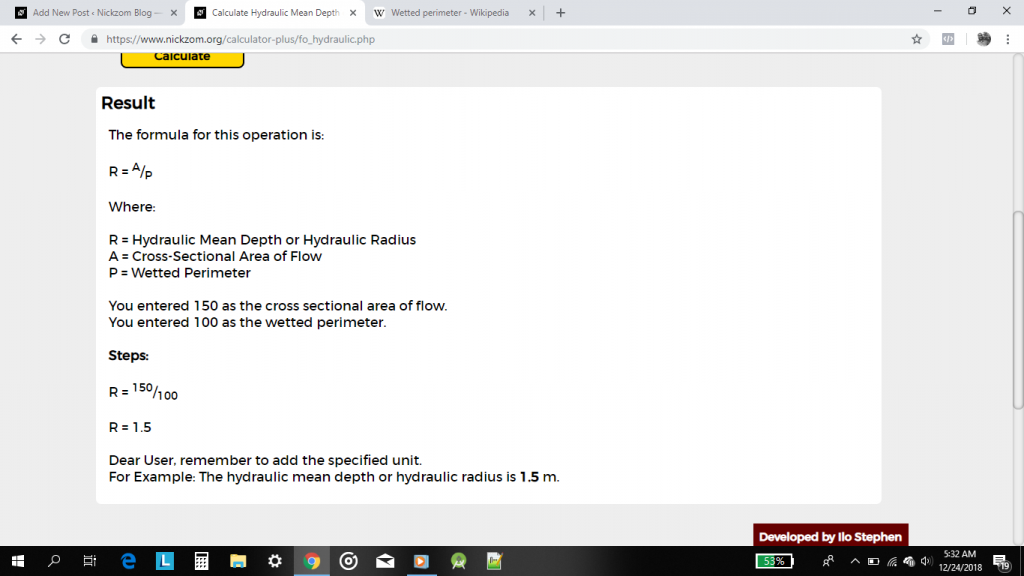# The Calculator Encyclopedia Calculates the Hydraulic Mean Depth or Hydraulic Radius of Flow in Open Channels | Fluid Mechanics

Hydraulic mean depth or hydraulic radius can be defined as the cross-sectional area of flow divided by wetted perimeter.

Wetted perimeter is the perimeter of the cross sectional area that is “wet”.
Hydraulic mean depth or hydraulic radius is a very important parameter in flow in open channels and fluid mechanics calculations.

The formula for computing hydraulic mean depth or hydraulic radius is:

R = A / P

Where:
R = Hydraulic Depth
A = Cross-sectional Area of Flow
P =  Wetted Perimeter

For Example:

Find the hydraulic mean depth or hydraulic radius of flow in an open channel where the cross-sectional area is 150 m2 and the wetted perimeter is 100 m.

From the example we can see that the cross-sectional area is 150 m2 and the wetted perimeter is 100 m.

R = 150 / 100
R = 1.5

Therefore, the hydraulic mean depth or hydraulic radius is 1.5 m.

Now, I would love to show you how to use Nickzom Calculator The Calculator Encyclopedia to get answers for your questions on hydraulic mean depth or hydraulic radius.

To have access to the calculator encyclopedia – Nickzom Calculator click on any of these links that suits best:
Web – https://www.nickzom.org/calculator-plus
Apple (Paid) – https://itunes.apple.com/us/app/nickzom-calculator/id1331162702?mt=8

it is preferable you obtain the professional version because that is what we are using in Nickzom Blog to showcase the powers of the calculator encyclopedia. Once you have got access to the app, proceed to the Calculator Map, then click on Mechanical under the Engineering section.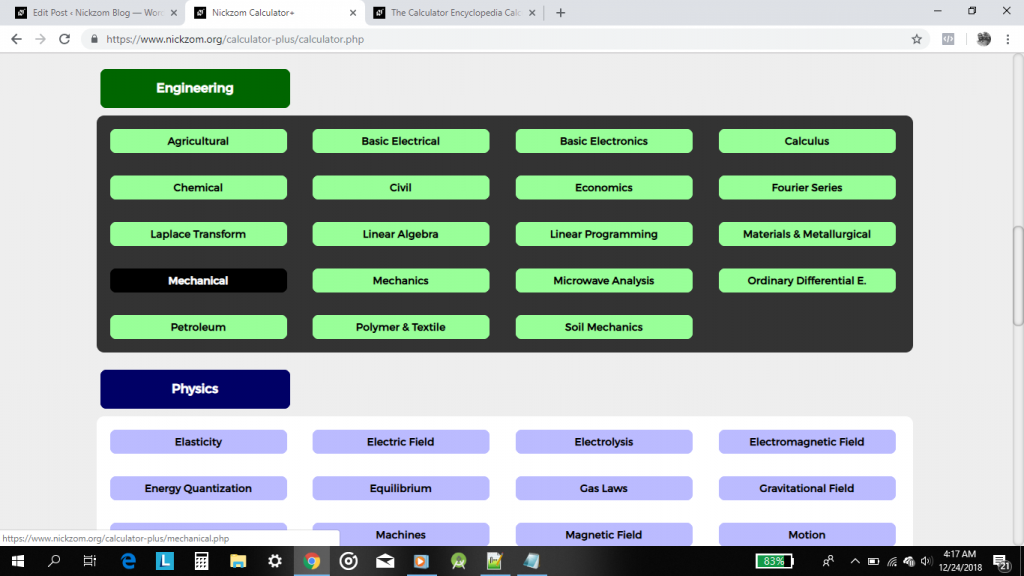Now, click on Fluid MechanicsNow, click on Flow in Open Channels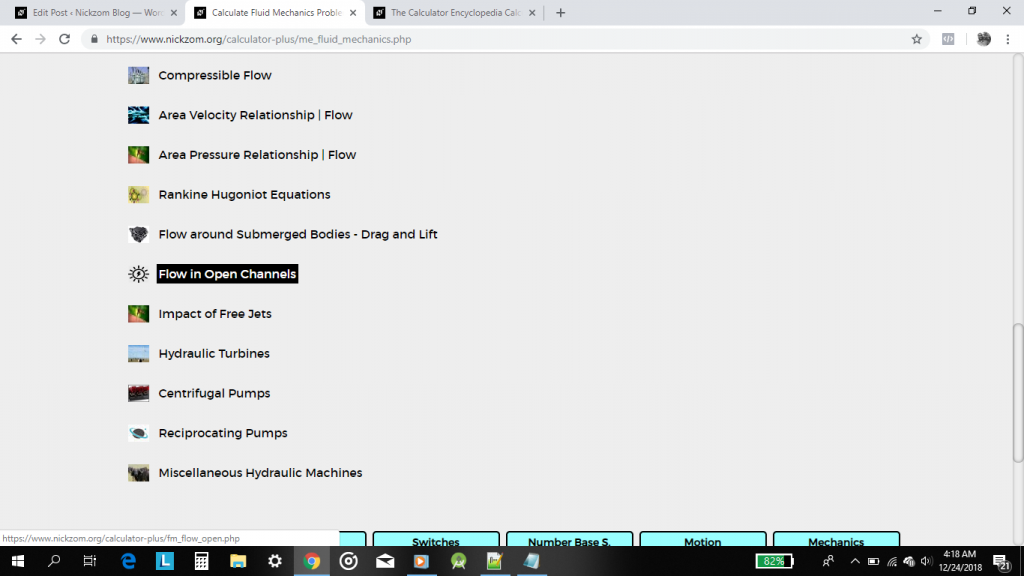Now, click on Hydraulic Depth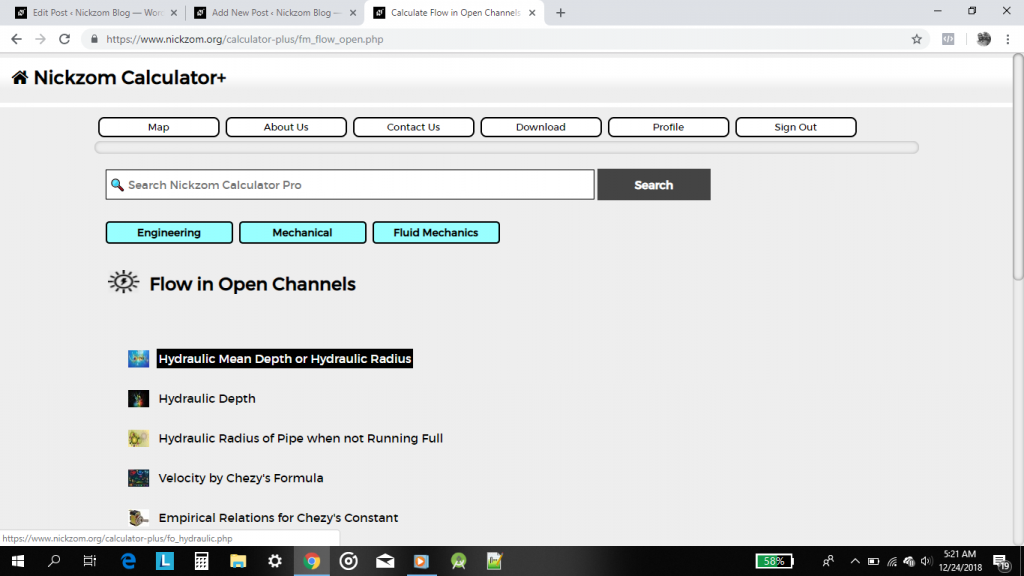A display of the parameters and input box needed to enter the values for getting the value of the hydraulic depth is now shown to you. Screenshot below!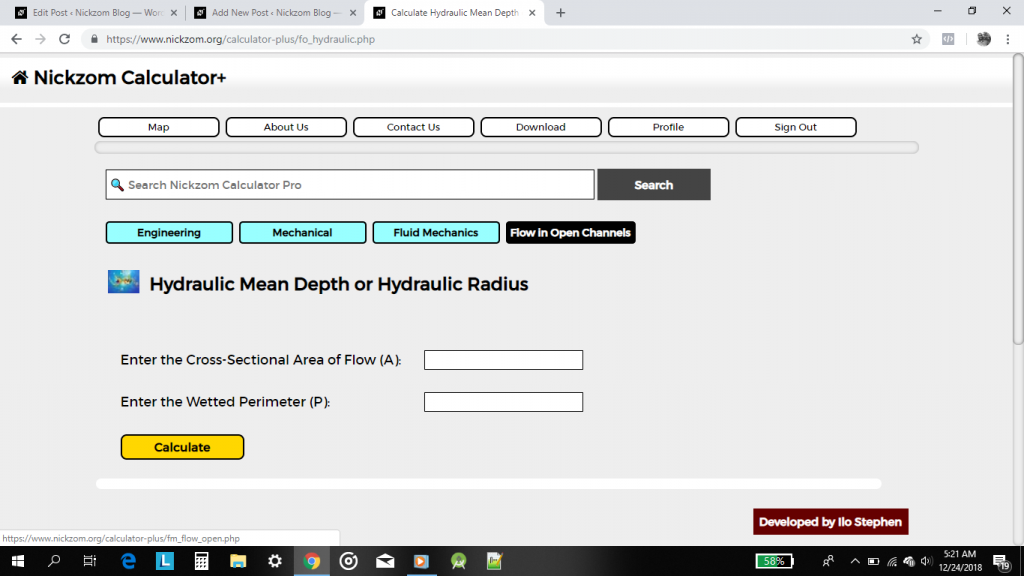From the example, the cross-sectional area of flow is 150 m2 and the wetted perimeter is 100 m.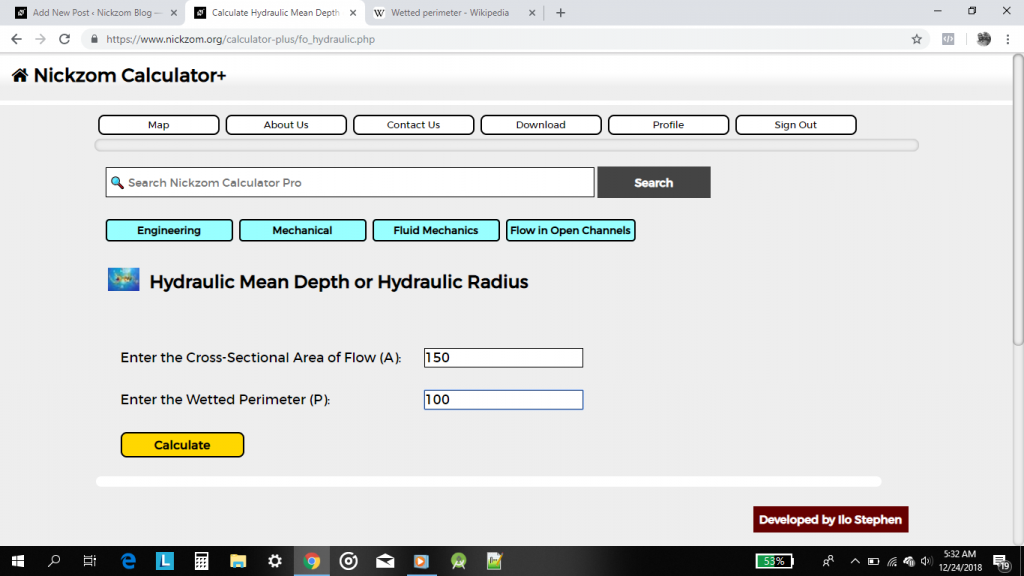Lastly, click on the Calculate button to get the hydraulic depth alongside the formula and workings. Screenshot Below!